# Auto-aim projectile stuff. (Read 7228 times)

Started by Maj', January 23, 2005, 09:58:18 pm

#### Maj'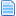##### Auto-aim projectile stuff.
#1  January 23, 2005, 09:58:18 pm
••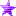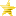When I was browsing through some of my old shit, I found this piece of code...

------------[piece of code]----------
projectile (helper)....

[State 0, ]
type = AngleSet
trigger1 = time = 0
value = (atan((p2bodydist y-86)/(ifelse(facing = 1, enemy, pos X - Pos X , Pos X - enemy, Pos X)))*(-180/Pi))

[State 0, ]
type = AngleDraw
trigger1 = 1

[State 8000, VelSet]
type = VelSet
trigger1 = time = 1
x = (90-(atan((p2bodydist y)/(ifelse(facing = 1, enemy, pos X - Pos X , Pos X - enemy, Pos X)))*(180/Pi)))/10
y = -(atan((p2bodydist y-86)/(ifelse(facing = 1, enemy, pos X - Pos X , Pos X - enemy, Pos X)))*(-180/Pi))/10
....
------------[/piece of code]----------

I thought it was interesting. I haven't seen much characters needing auto aiming projectiles though.
It's not really about the code, but about the formula. If you need more help or explenation on this, feel free to ask.

Sprites hosted at justnopoint

All my work is open source.
Last Edit: January 24, 2005, 12:02:21 am by Mr.E-ous

#### Bronko##### Re: Auto-aim projectile stuff.
#2  January 24, 2005, 12:57:41 am
••• Just a butcher on a mission.
If someone made an SSBM style samus...

#### Bahn##### Re: Auto-aim projectile stuff.
#3  April 13, 2005, 03:21:06 am
•Useful for replicating Shadow Lady's Galaxy Missile perhaps? How would I make it multiple projectiles?
Signatures should take up no more than 500x150 pixels onscreen, and images in signatures should total no more than 120kb (one hundred and twenty kilobytes) in size.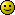#### BlackJack##### Re: Auto-aim projectile stuff.
#4  April 13, 2005, 03:23:53 am
••#### Winane##### Re: Auto-aim projectile stuff.
#5  April 13, 2005, 03:25:24 am
••• Tends to lose track of things a lot. :/
Still quite busy.

(Yes, I intend to deal with that stuff eventually, but kinda can't just yet, sorry. :/ )

#### BlackJack##### Re: Auto-aim projectile stuff.
#6  April 13, 2005, 03:39:33 am
••^-- yes, the opposite of what I said, and it's better.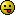Another method would be:

SMEE IIRC said:
Finds the X component of the vector Player, EnemyNear
;Formula to find the components of a given vector AB
;V(x) = B(x) - A(x)
;V(y) = B(y) - A(y)
[State 1640, X]
Type = VarSet
Trigger1 = 1
fvar(13) = (EnemyNear(0), Pos X - Pos X) * Facing ;Adjust the direction towards player facing

;Finds the Y component of the vector Player, EnemyNear
[State 1640, Y]
Type = VarSet
trigger1 = 1
fvar(14) = (EnemyNear(0), pos Y - Pos Y)

;Finds the vector length
;Formula of the length of a given vector AB
;|AB| = (AB(x)^2 + AB(y)^2)^1/2
[State 1640, Vector Length]
Type = VarSet
trigger1 = 1
fvar(15) = Exp(0.5*ln((fvar(13) * fvar(13))+(fvar(14) * fvar(14))))

;To find the normalized vector one has to use
;the following formula:
;Normal(AB) = V(AB)/|AB|
;So, you divide the X and Y components of one vector by it's length
;Obs:
;Normalized vector = The normalized vector of X is a vector in the same direction
;but with norm (length) 1.
;Now that we have the normalized vector, we multiply it by the desired absolute velocity,
;such as 5 pixels per frame.

;Projectile
[State 1640, Projectile]
Type = Projectile
trigger1 = animelem = 2
projanim = 1010
projhitanim = 1020
projremanim = 1020
projremovetime = 240
projpriority = 1
projsprpriority = 2
projid = 1000
offset = 0 ,-35
velocity = (Fvar(13)/fvar(15)) * 5.0, (FVar(14)/fvar(15)) * 5.0 ;Normalizes the components
;and multiply by the desired absolute velocity.
attr = S, NP
hitflag = MAF
damage = Floor(((3 + (3 * Random/1000.0)) * Fvar(28) + 0.5)), Floor(1 + (Random/1000.0) * Fvar(28) + 0.5)
animtype = Hard
guardflag = M
pausetime = 0,12
sparkno = 1
sparkxy = 26,0
hitsound = 5, 2
guardsound = 6, 1
guard.sparkno = 40
ground.type = High
ground.velocity = -2.4
ground.slidetime = 12
ground.hittime = 12
air.velocity = -2.4,-4.5
air.fall = 1
air.recover = 0
ignorehitpause = 1
getpower = 50, 75
givepower = 20, 30

#### Winane##### Re: Auto-aim projectile stuff.
#7  April 13, 2005, 05:39:08 am
••• Tends to lose track of things a lot. :/
Yeah, SMEE posted that in this thread.  That'll work if you'd rather use a Projectile than a Helper, and if you don't mind the projectile's animation not being rotated.  Though that can be worked around, by including a range of angled animations in the AIR file, and choosing which anim to use based on the calculated angle.
Still quite busy.

(Yes, I intend to deal with that stuff eventually, but kinda can't just yet, sorry. :/ )

#### Bronko##### Re: Auto-aim projectile stuff.
#8  April 13, 2005, 05:37:37 pm
••• Just a butcher on a mission.

#### Cyanide##### Re: Auto-aim projectile stuff.
#9  July 25, 2005, 06:58:12 am
••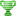• Legendary XIII
• I am the eye of the storm to come!
Gotta add something to this. I recently tried to use this code to make a missile face p2 after spinning in place for a few seconds and found it to be badly inaccurate (missile facing down original sprite) came out in all sorts of wacky angles. Anyway i changed the sum around a lot for the angledraw and this has given me good results over 5 tests

fvar(3) and var(2) have been set to p2dist Y and enemy, pos X respectively

Code:
`[state blah]type = anglesettrigger1 = 1value = 90+(atan((fvar(3))/(ifelse(facing = 1, var(2) - Pos X , pos X - Var(2))))*(-180/Pi))`

Also found that it must be facing P2 if it wasn't it inverted the direction ie missiles back facing me.

I think i'm gonna have to work on the velset bit too as that rocketed them off sideways at the speed of light.In M.U.G.E.N there is no magic button

They say a little knowledge is a dangerous thing, but it's not one half so bad as a lot of ignorance.